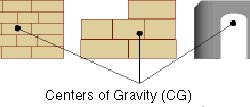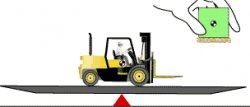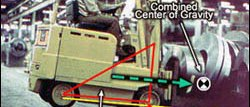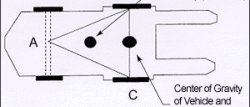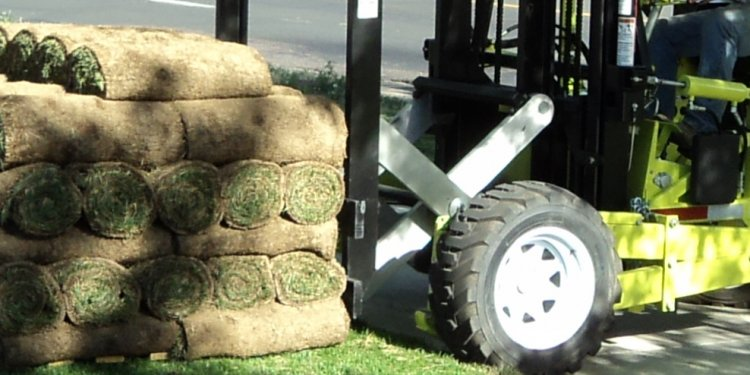# Forklift on Truck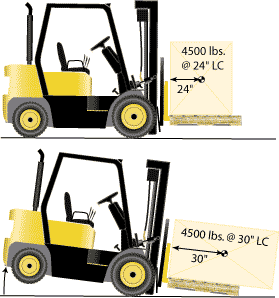Figure 2. The same 4500 pounds weight loaded properly (top) will exceed the rated capacity of 4500 pounds if the rectangular box is positioned lengthwise (bottom).
Load weight, weight distribution, size, shape, and position are key factors affecting the stability of the forklift. Forklifts are designed to carry a capacity load at a standard load center, commonly 24 inches. This means that the forklifts capacity was determined as if the load were a cube whose weight is evenly distributed (i.e., whose center of gravity is exactly in the center of the cube) and which is resting on a standard pallet having dimensions of 48 inches by 48 inches. With such a load, the horizontal distance from the center of the load to the vertical part of the forks would be 24 inches. Of course, most loads are not perfectly shaped cubes having their center of gravity exactly in the middle of the cube. To the extent that the load differs from this theoretical load  such as if it is irregularly shaped, has unbalanced weight distribution, or is not centered on the forks  the capacity may be reduced.

Potential Hazards:

While arranging a load, be aware of the following:

• tipover
• Loss of steering control (Shifting too much weight forward raises the rear wheels.)
• Collision
Requirements and Recommended Practices:
• Do not exceed the capacity of the forklift that appears on the forklift's data plate (sometimes called the "nameplate"). If the load is oversized, irregularly shaped, or loaded incorrectly, the actual load center distance could exceed the stated load center distance, causing the truck's capacity to be exceeded. (Figure 2).
• Always minimize the distance from the front wheels to the load center. Load a large rectangular box widthwise across the forks of the truck as in Figure 2. Placing a large rectangular load lengthwise causes the load center to shift forward further away from the front wheels, exceeding the truck's capacity and lifting the rear wheels off the ground.

Figure 3. The heaviest weight should be loaded as close to the masts as possible.

• Load as close to the front wheels as possible to minimize the load center distance. Load the heaviest part toward the mast. (Figure 3)
• Position the load in a way that will shorten the load center distance.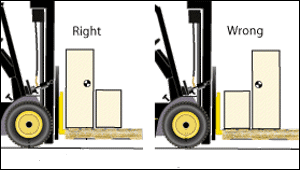Figure 4. Improperly distributed loads may tip the forklift if the operator exceeds the stated capacity of the truck. This forklift can carry 4, 000 pounds at a 24 inches load center, but only 2, 666 pounds at a 36 inches load center.
Requirements and Recommended Practices:
• If the stated load center is exceeded, compensate by reducing the weight of the load.
• Consult the forklift manufacturers instructions when handling large or unusually configured loads.
• Tip: Use field calculations to estimate the reduced lifting capacity if manufacturers instructions are not available. This calculation method will not produce exact load reduction figures. Use this method only as a guideline. The forklift manufacturer is the source of more precise information.
Field Calculation of Safe Load Capacity

Assume a situation where a forklift truck that has a 5, 000 pound capacity at a 24 inch load center needs to handle a load whose center is 28 inches from the front face of the forks in the horizontal direction. The first thing to recognize is that the actual load center distance of 28 inches exceeds the standard load center distance of 24 inches on which the 5000 pound capacity is based, so the safe load capacity is actually less than 5000 pounds.

To estimate the truck's safe load capacity at a 28-inch load center, take the rated load center and divide it by the actual load center. Then multiply this number by the stated capacity to get the new approximate safe load capacity:

24 in/28 in x 5, 000 lb = 4, 285 lb (approximate safe load capacity)

Using the example in Figure 4, take the stated standard load center of 24 inches and divide it by the actual load center of 36 inches. Multiply this number by the stated capacity of 4, 000 lb to get the new approximate safe load capacity:

24 in/36 in x 4, 000 lb = 2, 666 (approximate safe load capacity)

 Figure 5. Lifting a 5 lb box directly up has the effect of lifting 5 lbs. Figure 6. As the distance increases from the shoulder, the moment, or apparent weight increases so that a 5 pound box seems to weigh 12 pounds. View Animation
The way in which weight is distributed changes the amount of weight the lift truck will safely carry. You can experience this for yourself by doing the following activity: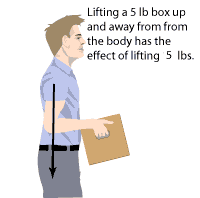Lift a 5 pound box. As you extend your arms, the center of the boxs weight moves a greater distance from your body, so the box feels heavier and you will tend to fall forward. The same idea of increasing the load center distance applies to a playground see-saw: the farther you sit from the middle, the more you increase the load center distance and the more force you put on that end. The same principleincreasing the load center distancecan cause a forklift to tipover.

When the load center distance increases, it is actually increasing something called the "Load Moment":

Load Moment is the product of the object's weight multiplied by the objects distance from the fulcrum, which is a fixed point that acts as the pivot point. On a sit-down counterbalanced forklift, the fulcrum or pivot point is the axle of the front wheels. It is this product, or Load Moment, which determines how much overturning force is being applied to the forklift.

Load Moment = Weight X Distance

If a load is 60 inches long (30-inch load center) then the maximum that this load can weigh is:

72, 000 inch-pounds / 30 in-load center = 2, 400 pounds

Figure 7. Improperly distributed loads may tip the forklift if the maximum load moment is exceeded.

Potential Hazards:

While carrying a load near the maximum allowable capacity, be aware of the following:

• Danger of tipover
• Danger of being struck by falling load
Requirements and Recommended Practices:
• Calculate a maximum allowable load moment to determine whether an unusual load, such as one that is longer than 48 inches (i.e., the load center distance would be greater than 24 inches) or that has an offset center of gravity (i.e., uneven weight distribution) can be handled safely.
• Minimize the load center distance measured from the back of the forks to the center of the load. This allows the forklift to carry more weight.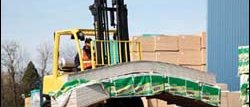As illustrated in Figure 7, a truck that has a 4, 500 pound capacity at a 24-inch load center will tipover if a 60-inch load is positioned lengthwise. Positioning the load in this way increases the load center distance to 30 inches and increases the load moment by 27, 000 inch-pounds.

In Figure 7 the forklift safely carries the 4, 500 pound load at a load center distance of 24 inches, but tips over when the load center increases to 30 inches. Here's the calculation: 30 inches X 4, 500 pounds = 135, 000 inch-pounds
24 inches X 4, 500 pounds = 108, 000 inch-pounds The load moment is increased by 27, 000 inch-pounds.

If the load center distance is 30 inches, the only way to keep the maximum allowable load moment within 108, 000 inch-pounds is to limit the weight of the load to 3600 pounds:

30 inches X 3600 pounds = 108, 000 inch-pounds

• Use extra caution when handling extra heavy loads that may approach the truck's maximum capacity. For example, when handling a maximum load, the load should be carried at the lowest position possible, the truck should be accelerated slowly and evenly, and the forks should be tilted forward cautiously. However, there is no one rule for all situations.
• Maintain control of the vehicle at all times. The operator is responsible for handling the truck. Drive slower when carrying a load near the maximum allowable.
• Do not exceed the stated capacity of your truck. Know its mechanical limits.
 Figure 8. Stability Triangle formed by connecting the three support points of a powered industrial truck's suspension system.
Understanding why forklifts tipover and why loads become unstable and fall is very important to preventing accidents. Important factors that affect a forklift's balance are:
Center of Gravity

While operating a forklift, be aware of the following:

Figure 9. Use special care when carrying a wide load. Turn slowly to prevent the load from shifting.

Requirements and Recommended Practices:
• Keep the center of gravity of the load as near as possible to the center going horizontally across the forks.
• Keep the center of gravity of the load as near to the front wheels as possible.
Shifting Center of Gravity

Figure 10. The Center of Gravity (CG) is in the center of a symmetrical load but is off center in an irregular load. In the third example, the CG is outside the boundaries of the object.

Figure 11. A 4, 000 pound truck is balanced by a 4, 000 pound load.

Figure 12. Notice the center of gravity of the load and truck system shift forward toward the front wheels as the load is engaged.
View Animation

All objects have a specific center of gravity. Gravity is a force that always pulls objects toward the earth's core. Center of gravity means the point on an object at which all of the objects weight is concentrated and all of the parts balance each other. For symmetrical loads, the center of gravity is at the middle of the load in terms of the loads length, width and height. (Figure 10). Since the capacity of the forklift is based on the assumption of a cube having the center of gravity in the middle, the shape and position of the actual load are key factors when determining whether a load can be carried safely.